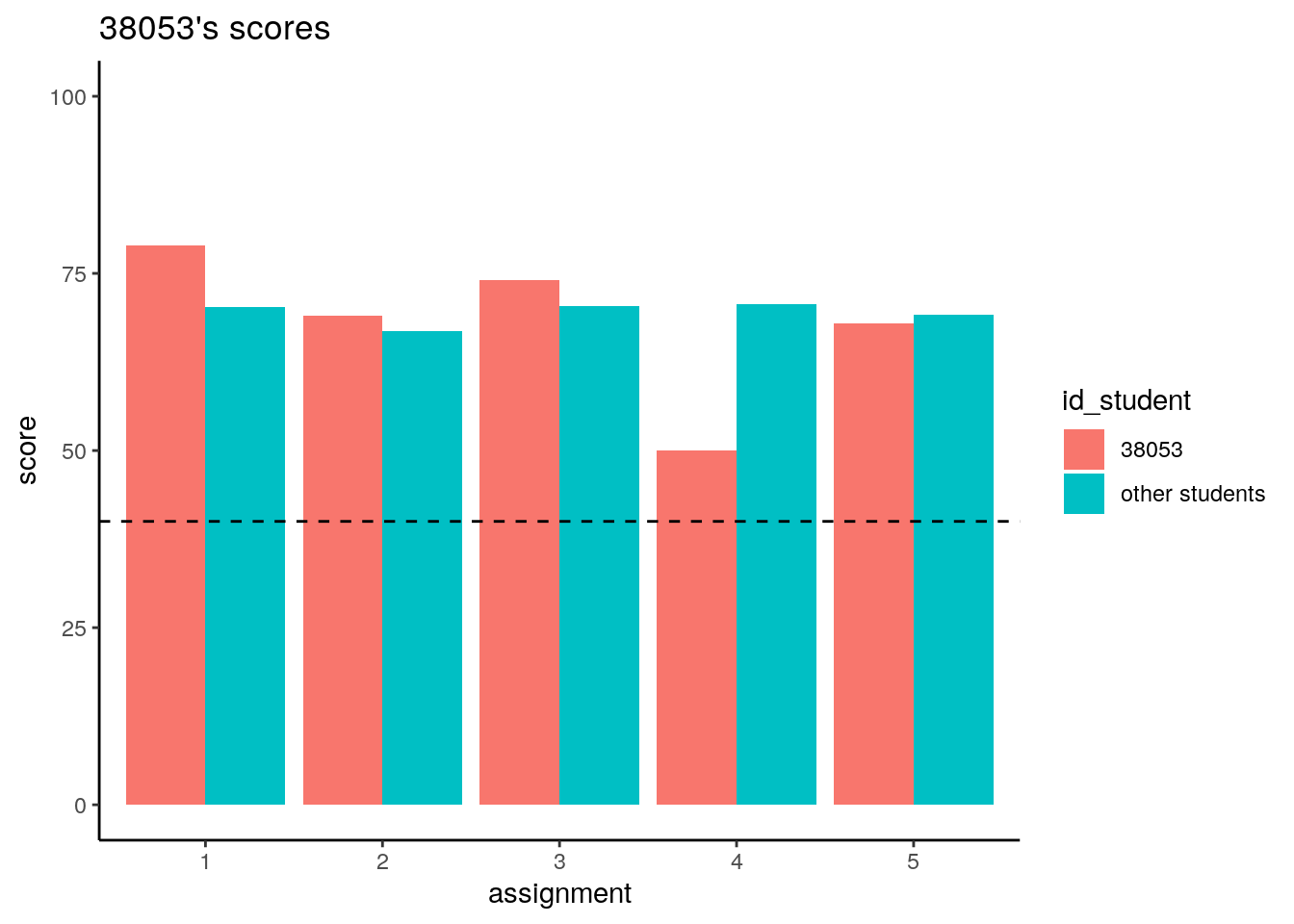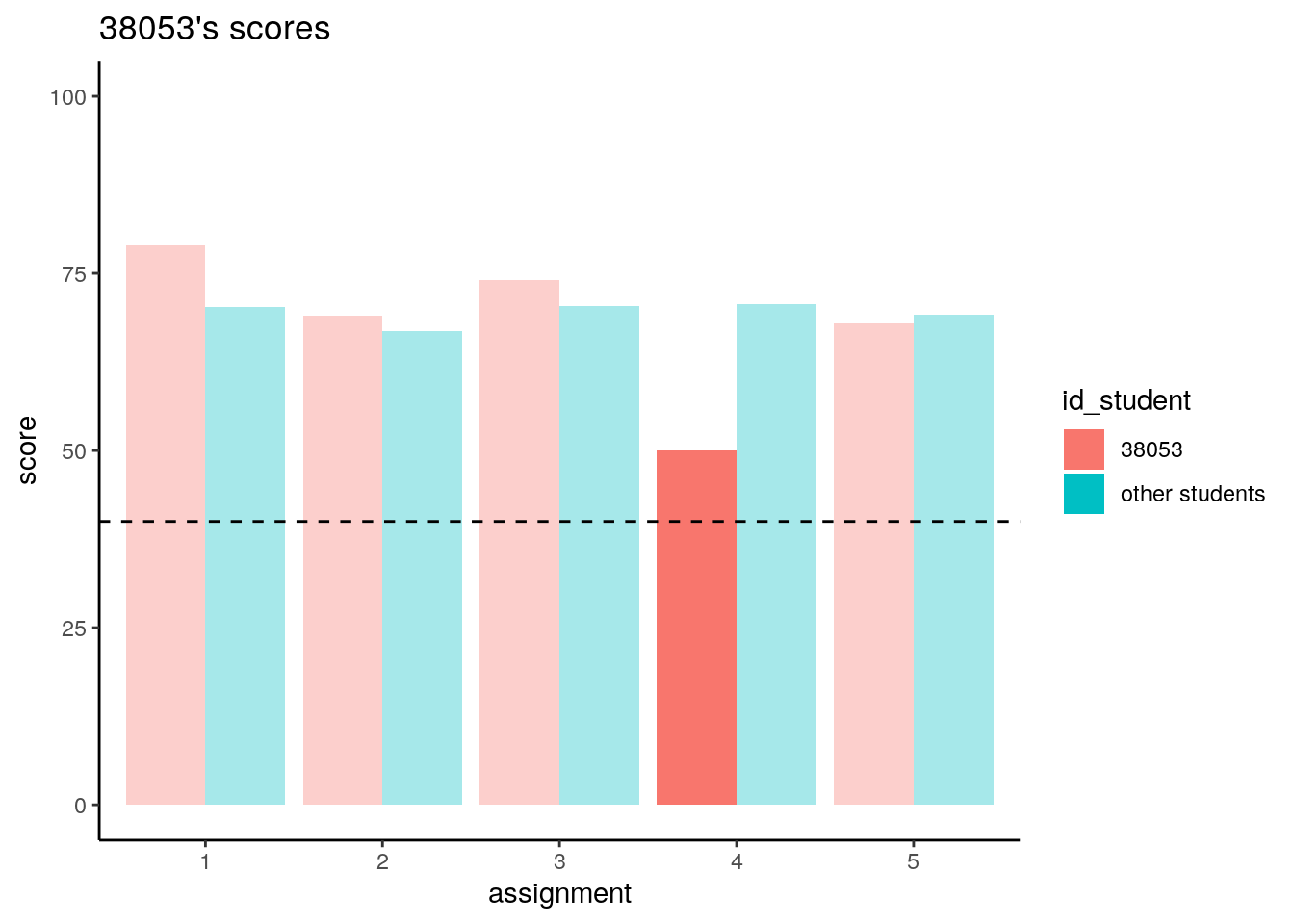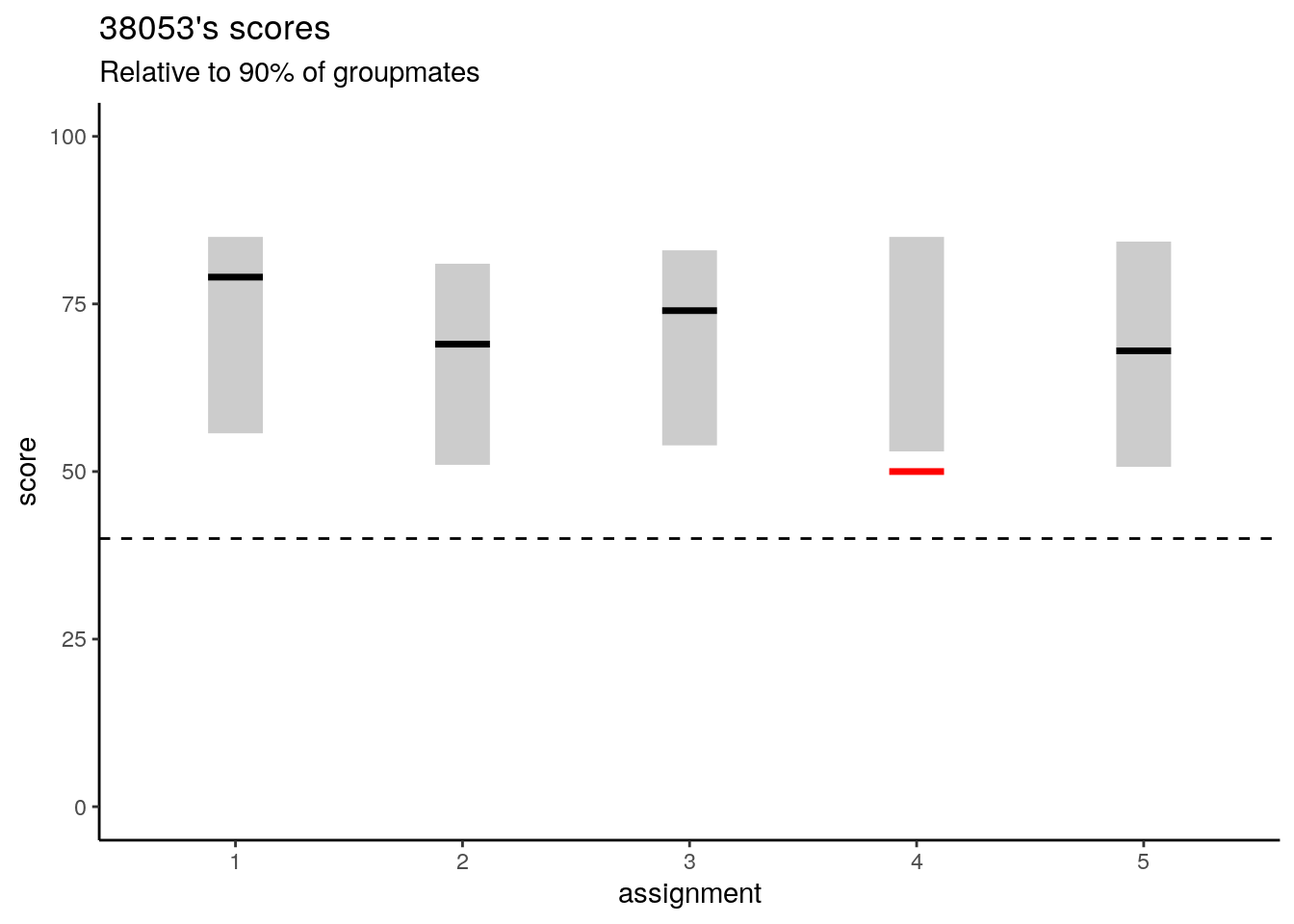# 3 Dashboard for students

``library(tidyverse)``

Let’s construct a prototype of dashboard for one of our students.

Discussion:
- How to show student’s perfomance?
- Should we show students their predicted chances for success?

On the graph below we show the score for each assesment aside with the average score of other students.

``````ggplot() +
geom_bar(data = dfp, aes(x = assignment, y = score, fill = id_student), stat = "identity", position = "dodge") +
geom_hline(yintercept = 40, color = "black", linetype = "dashed") +
ggtitle(str_c(user, "\'s scores")) +
theme_classic() +
ylim(0, 100)``````We can highlight some of the student’s results which have notable differences with the average score. Whether it will be usefull or motivating for the student? Maybe we should show that graph to the teacher or assistant (in cases where an individual supervisement is possible).

``````ggplot() +
geom_bar(data = dfp, aes(x = assignment, y = score, fill = id_student, alpha = diff_score_highlight), stat = "identity", position = "dodge") +
scale_alpha_manual(values = c("TRUE" = 1, "FALSE" = 0.35), guide = "none") +
geom_hline(yintercept = 40, color = "black", linetype = "dashed") +
ggtitle(str_c(user, "\'s scores")) +
theme_classic() +
ylim(0, 100)``````Alternative way is to show student’s grade in the context of the range of other’s grades.

``````ggplot() +
geom_segment(data = dfp, aes(x = assignment, xend = assignment, y = score10, yend = score90), size = 10, alpha = 0.2) +
geom_segment(data = dfp, aes(x = assignment, xend = assignment, y = score-0.5, yend = score+0.5, color = highlight), size = 10) +
scale_color_manual(values = c("no" = "black", "low" = "red", "high" = "green"), guide = "none") +
geom_hline(yintercept = 40, color = "black", linetype = "dashed") +
ylim(0, 100) +
ylab("score") +
theme_classic() +
ggtitle(label = str_c(user, "\'s scores"), subtitle = "Relative to 90% of groupmates")``````Discussion:
- What is the relation between the difference of assignment submission to deadline and the grade, in your experience?
- How could we show that relation to students? What might be our recommendations?